# Explain The Logic NOR Gate and Its operation and How It Works as an Universal Gate

## NOR Gate

The term NOR is a contraction of NOT-OR and implies an OR function with an inverted output. The output of this gate is high, only when all input voltages are low. A standard logic symbol for a 2-input NOR gate and the equivalent OR gate followed by an INVERTER are shown in fig 1 .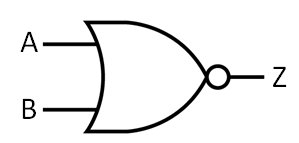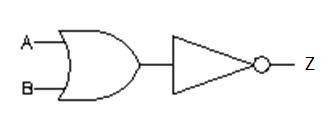Fig.1 (a)                                                                        Fig 1(b)

The standard symbol for the NOR gate is the same as the OR gate symbol except that it has a small circle on the output. This small circle represents the inversion operation. Thus, the NOR gate operates like an OR gate followed by an INVERTER , so that the circuits shown in fig 1(a) and (b) equivalent.

The operation of this gate can be described as below:

The output of the OR gate, Z’ can be written as :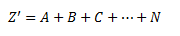and the output of the NOT gate can be written as :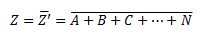The logical operation represented by the above equation is known as the NOR operation.

### Truth Table of NOR Gate

The truth table from 2-input NOR gate is given in Table 1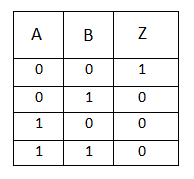Table 1

From table 1 we find that NOR gate output is the exact inverse of the OR-gate output for all possible input condition. Whereas an OR-gate output goes high when any input is high, the NOR gate output goes low when any input is high. This same operation can be extended to NOR gates with more than two inputs.

The logic equation of NOR gate is given asand is read as ‘Z equals NOT (A OR B)’.

### Transistor NOR Gate

A simple 2-input logic NOR gate can be constructed using RTL Resistor-transistor switches connected together as shown below with the inputs connected directly to the transistor bases.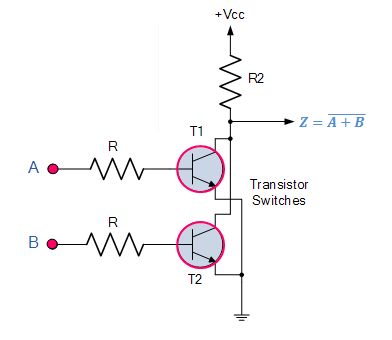Fig 2

As seen from the fig 2, the output Z is 1 only when both transistors are cutoff i.e. when A = 0 and B = 0. For any other condition of input such as A = 1 , B = 0, or A = 0, B = 1 or A = 1, B = 1 one or both transistors operate in saturation and as result the output Z is low (i.e.0).

### NOR Gate equivalent using AND Gate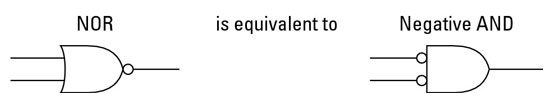Fig 3

In fig. 3 AND gate with input signals inverted is shown.

It also acts as a NOR gate.

If we look at table 2 (truth table for AND gate with its inverted inputs) we find that , it is same as table 1 (truth table for NOR gate).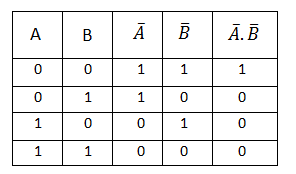Table 2

So, AND gate with its inputs inverted can use used as NOR gate.

### NOR  as Universal Gate

Like the NAND gate discussed in our previous tutorial, the NOR gate can also be classed as a “Universal” type gate.

NOR gates can be used to produce any other type of logic gate function just like the NAND gate .

It is interesting to note that the three basic logic operations NOT, OR and AND can be performed by using only the NOR gates .

NOT logic operation can be performed by typing together the two inputs, as shown in fig 4 .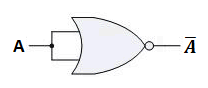fig 4

OR logic operation can be performed by using three NOR gates, as shown in fig 5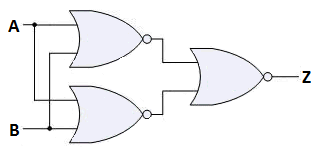Fig.5

The final output is inverted and is given as  Z = A + B , which is a logic function of a normal OR gate.

AND operation can be performed by using three NOR gates, as illustrated in fig 6 .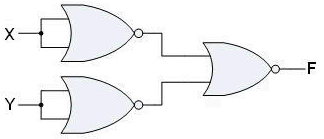fig 6

The inputs have, thus, been inverted before they are applied to the NOR gate. The output (A + B)’ equals A.B. It can be proved with the help of De Morgan’s theorem.

### Standard Package

Fig.7 depicts the pinout diagram of an IC 7402, a TTL quad 2 input NOR gate.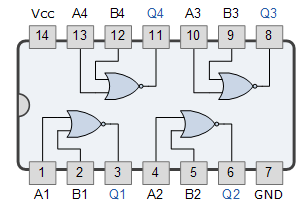Fig 7

This IC contains for 2-input NOR gates inside a 14-pin dual-in-line package(DIP).

### Pulsed Operation of NOR Gate

Fig 8 shows the waveform of two inputs A and B to a NOR gate.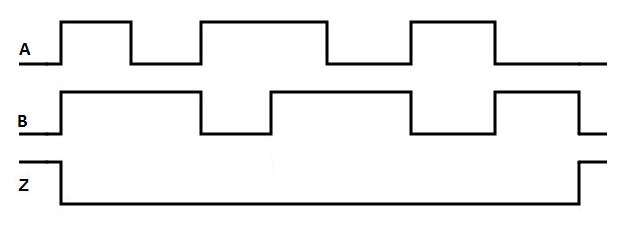Fig 8

It can be noticed from the above waveforms that the output is low when either of the two inputs A or B is high and the output is high when both of the inputs are low.